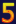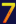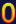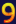- Mahatma Gandhi
More pages: 1 2
Monday, April 27, 2009 | Permalink

The depth buffer is increasingly being used for more than just hidden surface removal. One of the more interesting uses is to find the position of already rendered geometry, for instance in deferred rendering, but also in plain old forward rendering. The easiest way to accomplish this is something like this:

float4 pos = float4(In.Position.xy, sampled_depth, 1.0);
float4 cpos = mul(pos, inverse_view_proj_scale_bias);
float3 world_pos = cpos.xyz / cpos.w;

The inverse_view_proj_scale matrix is the inverse of the view_proj matrix multiplied with a scale_bias matrix that brings the In.Position.xy from [0..w, 0..h] into [-1..1, -1..1] range.

The same technique can of course also be used to compute the view position instead of the world position. In many cases you're only interested in the view Z coordinate though, for instance for fading soft particles, fog distance computations, depth of field etc. While you could execute the above code and just use the Z coordinate this is more work than necessary in most cases. Unless you have a non-standard projection matrix you can do this in just two scalar instructions:

float view_z = 1.0 / (sampled_depth * ZParams.x + ZParams.y);

ZParams is a float2 constant you pass from the application containing the following values:

ZParams.x = 1.0 / far - 1.0 / near;
ZParams.y = 1.0 / near;

If you're using a reversed projection matrix with Z=0 at far plane and Z=1 at near plane you can just swap near and far in the above computation.

Name

Comment

Enter the code belowGreg
Friday, May 1, 2009